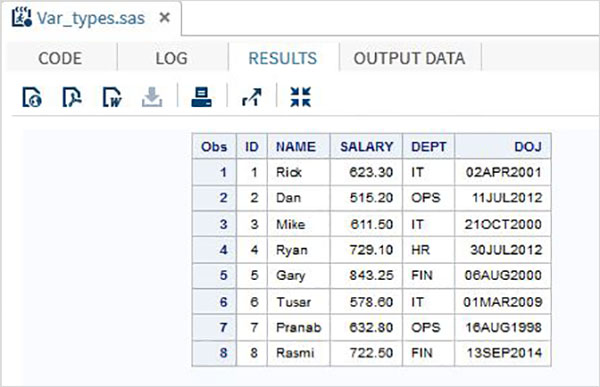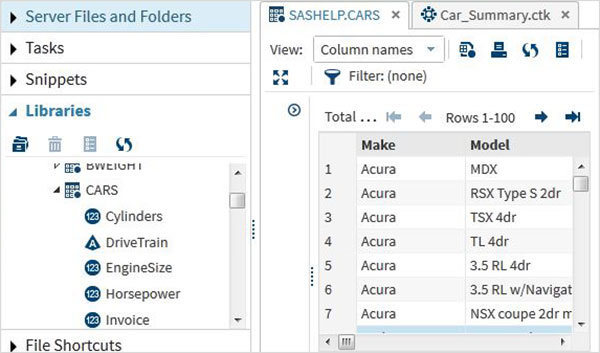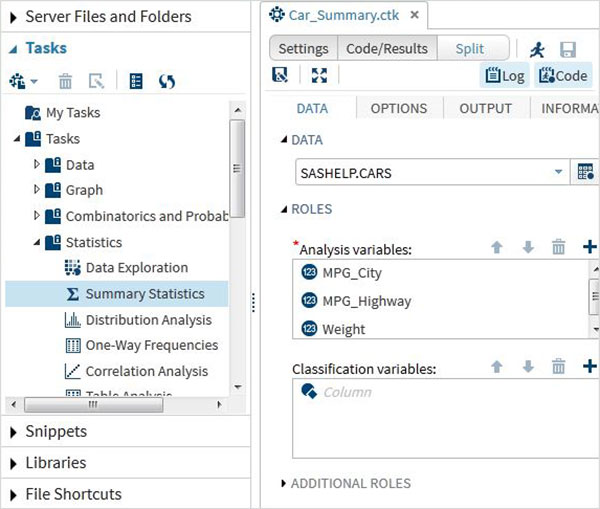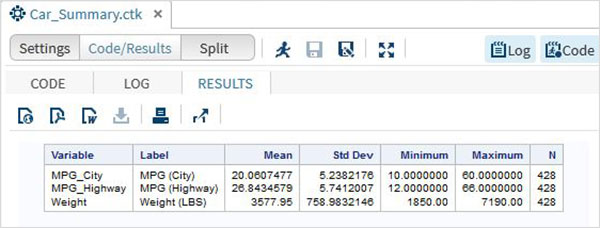# SAS - Variables

In general variables in SAS represent the column names of the data tables it is analysing. But it can also be used for other purpose like using it as a counter in a programming loop. In the current chapter we will see the use of SAS variables as column names of SAS Data Set.

## SAS Variable Types

SAS has three types of variables as below −

### Numeric Variables

This is the default variable type. These variables are used in mathematical expressions.

### Syntax

```INPUT VAR1 VAR2 VAR3; 		#Define numeric variables in the data set.
```

In the above syntax, the INPUT statement shows the declaration of numeric variables.

### Example

```INPUT ID SALARY COMM_PERCENT;
```

### Character Variables

Character variables are used for values that are not used in Mathematical expressions. They are treated as text or strings. A variable becomes a character variable by adding a \$ sing with a space at the end of the variable name.

### Syntax

```INPUT VAR1 \$ VAR2 \$ VAR3 \$; 	#Define character variables in the data set.
```

In the above syntax, the INPUT statement shows the declaration of character variables.

### Example

```INPUT FNAME \$ LNAME \$ ADDRESS \$;
```

### Date Variables

These variables are treated only as dates and they need to be in valid date formats. A variable becomes a date variable by adding a date format with a space at the end of the variable name.

### Syntax

```INPUT VAR1 DATE11. VAR2 MMDDYY10. ; #Define date variables in the data set.
```

In the above syntax, the INPUT statement shows the declaration of date variables.

### Example

```INPUT DOB DATE11. START_DATE MMDDYY10. ;
```

## Use of Variables in SAS Program

The above variables are used in SAS program as shown in below examples.

### Example

The below code shows how the three types of variables are declared and used in a SAS Program

```DATA TEMP;
INPUT ID NAME \$ SALARY DEPT \$ DOJ DATE9. ;
FORMAT DOJ DATE9. ;
DATALINES;
1 Rick 623.3 IT 02APR2001
2 Dan 515.2 OPS 11JUL2012
3 Michelle 611 IT 21OCT2000
4 Ryan 729 HR 30JUL2012
5 Gary 843.25 FIN 06AUG2000
6 Tusar 578 IT 01MAR2009
7 Pranab 632.8 OPS 16AUG1998
8 Rasmi 722.5 FIN 13SEP2014
;
PROC PRINT DATA = TEMP;
RUN;
```

In the above example all the character variables are declared followed by a \$ sign and the date variables are declared followed by a date format. The output of the above program is as below.## Using the Variables

The variables are very useful in analysing the data. They are used in expressions in which the statistical analysis is applied. Let’s see an example of analysing the built-in Data Set named CARS which is present under Libraries → My Libraries → SASHELP. Double click on it to explore the variables and their data types.Next we can produce a summary statistics of some of these variables using the Tasks options in SAS studio. Go to Tasks -> Statistics -> Summary Statistics and double click it to open the window as shown below. Choose Data Set SASHELP.CARS and select the three variables - MPG_CITY, MPG_Highway and Weight under the Analysis Variables. Hold the Ctrl key while selecting the variables by clicking. Click run.Click on the results tab after the above steps. It shows the statistical summary of the three variables chosen. The last column indicates number of observations (records) used in the analysis.